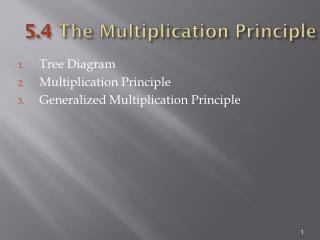# 5.4 The Multiplication Principle - PowerPoint PPT PresentationDownload Presentation5.4 The Multiplication Principle

5.4 The Multiplication Principle
Download Presentation## 5.4 The Multiplication Principle

- - - - - - - - - - - - - - - - - - - - - - - - - - - E N D - - - - - - - - - - - - - - - - - - - - - - - - - - -
##### Presentation Transcript

1. 5.4 The Multiplication Principle • Tree Diagram • Multiplication Principle • Generalized Multiplication Principle

2. Tree Diagram A tree diagram is a graph showing possibilities for an event having choices along the way. Suppose a rat in a maze starts at point A. There are five possible routes to get from point A to point B and 3 possible routes to get from point B to the final destination, point C. Represent the possible choices using a tree diagram.

3. Tree Diagram (2) Possible paths: 1a, 1b, 1c 2a, 2b, 2c 3a, 3b, 3c 4a, 4b, 4c 5a, 5b, 5c Total possible paths = 15

4. Multiplication Principle Multiplication Principle Suppose that a task is composed of two consecutive operations. If operation 1 can be performed in m ways and, for each of these, operation 2 can be performed in n ways, then the complete task can be performed in mn ways.

5. Example Multiplication Principle • Use the multiplication principle to determine the number of different paths a rat can take going from point A to point C if there are five possible routes to get from point A to point B and 3 possible routes to get from point B to point C. • There are 5 possible routes for task 1 and 3 possible routes for task 2 so there are 53 = 15 possible routes. This agrees with the tree diagram drawn previously.

6. Generalized Multiplication Principle Generalized Multiplication Principle Suppose that a task is composed of t operations performed consecutively. Suppose operation 1 can be performed in m1 ways; for each of these, operation 2 can be performed in m2 ways; for each of these, operation 3 can be performed in m3 ways; and so forth. Then the complete task can be performed in m1m2 m3…mt ways.

7. Example Generalized Principle • A corporation has a board of directors consisting of 10 members. The board must select from among its members a chairperson, vice chairperson, and secretary. In how many ways can this be done?

8. Example Generalized Principle (2) • The operations are: • 1. Select chairperson - there are 10 ways (people) to do this. • 2. Select a vice chairperson - there are 9 ways (9 people left after chairperson is chosen) to do this. • 3. Select a secretary - there are 8 ways to do this. • Total number of ways to complete task is 1098 = 720 ways.

9. Summary Section 5.4 • The multiplication principle states that the number of ways a sequence of several independent operations can be performed is the product of the number of ways each individual operation can be performed.